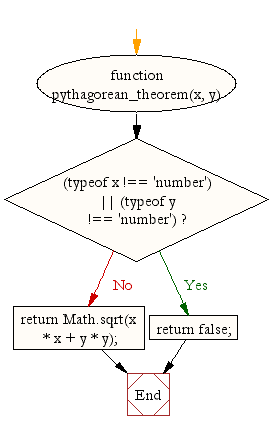# JavaScript : Pythagorean function in JavaScript

## JavaScript Math: Exercise-19 with Solution

Create a Pythagorean function in JavaScript.

Note : The Pythagorean Theorem tells us that the relationship in every right triangle is : c2 = a2 + b2, where c is the hypotenuse and a, b are two legs of the triangle.

Test Data:
console.log(pythagorean_theorem(2, 4));
console.log(pythagorean_theorem(3, 4));
Output :
4.47213595499958
5

Sample Solution:-

HTML Code:

``````<!DOCTYPE html>
<html>
<meta charset="utf-8">
<title>Pythagorean Theorem</title>
<body></body>
</html>
```
```

JavaScript Code:

``````function pythagorean_theorem(x, y) {
if ((typeof x !== 'number') || (typeof y !== 'number'))
return false;
return Math.sqrt(x * x + y * y);
}

console.log(pythagorean_theorem(2, 4));
console.log(pythagorean_theorem(3, 4));
```
```

Sample Output:

```4.47213595499958
5
```

Flowchart:Live Demo:

See the Pen javascript-math-exercise-19 by w3resource (@w3resource) on CodePen.

Improve this sample solution and post your code through Disqus

What is the difficulty level of this exercise?

Test your Programming skills with w3resource's quiz.

﻿